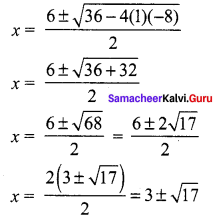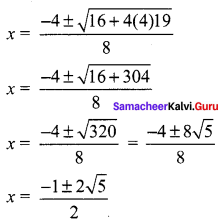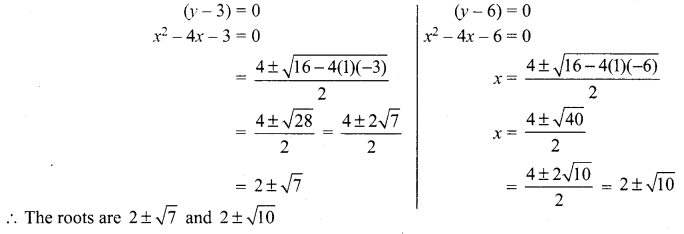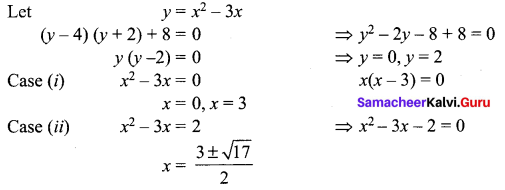# Samacheer Kalvi 12th Maths Solutions Chapter 3 Theory of Equations Ex 3.4

## Tamilnadu Samacheer Kalvi 12th Maths Solutions Chapter 3 Theory of Equations Ex 3.4

Question 1.
Solve:
(i) (x – 5) (x – 7) (x + 6) (x + 4) = 504
(ii) (x – 4) (x – 7) (x – 2) (x + 1) = 16
Solution:
(i) (x – 5) (x + 4) (x – 7) (x + 6) = 504
(x2 – x – 20) (x2 – x – 42) = 504
Let y = (x2 – x)
(y – 20) (y – 42) = 504
⇒ y2 – 42y – 20y + 840 = 504
⇒ y2 – 62y + 336 = 0
⇒ (y – 56) (y – 6) = 0
⇒ (y – 56) = 0 or (y – 6) = 0
⇒ x2 – x – 56 = 0 or x2 – x – 6 = 0
⇒ (x – 8) (x + 7) = 0 or (x – 3) (x + 2) = 0
⇒ x = 8, -7 or x = 3, -2
The roots are 8, -7, 3, -2

(ii) (x – 4) (x – 7) (x – 2) (x + 1) = 16
⇒ (x – 4) (x – 2) (x – 7) (x + 1) = 16
⇒ (x2 – 6x + 8) (x2 – 6x – 7) = 16
Let x2 – 6x = y
(y + 8)(y – 7) = 16
⇒ y2 – 7y + 8y – 56 – 16 = 0
⇒ y2 + y – 72 = 0
⇒ (y + 9) (y – 8) = 0
y + 9 = 0
x2 – 6x + 9 = 0
(x – 3)2 = 0
x = 3, 3
or
y – 8 = 0
x2 – 6x – 8 = 0The roots are 3, 3, 3 ±√17Question 2.
Solve : (2x – 1) (x + 3) (x – 2) (2x + 3) + 20 = 0.
Solution:
(2x – 1) (2x + 3) (x + 3) (x – 2) + 20 = 0
⇒ (4x2 + 6x – 2x – 3) (x2 – 2x + 3x – 6) + 20 = 0
⇒ (4x2 + 4x – 3) (x2 + x – 6) + 20 = 0
⇒ [4(x2 + x) – 3] [x2 + x – 6] + 20 = 0
Let y = x2 + x
⇒ (4y – 3) (y – 6) + 20 = 0
⇒ 4y2 – 24y – 3y + 18 + 20 = 0
⇒ 4y2 – 27y + 38 = 0
⇒ (4y – 19) (y – 2) = 0
(4y – 19) = 0
4(x2 + x) – 19 = 0
4x2 + 4x – 19 = 0or
(y – 2) = 0
x2 + x – 2 = 0
(x + 2) (x – 1) = 0
x = -2, +1
The roots are -2, 1, $$\frac{-1 \pm 2 \sqrt{5}}{2}$$

### Samacheer Kalvi 12th Maths Solutions Chapter 3 Theory of Equations Ex 3.4 Additional Questions

Questions 1.
Solve (x – 3) (x – 6) (x – 1) (x + 2) + 54 = 0.
Solution:
(x – 3) (x – 1) (x – 6) (x + 2) + 54 = 0
(x2 – 4x + 3) (x2 – 4x – 12) + 54 = 0
Put x2 – 4x = y
(y + 3)(y – 12) + 54 = 0
y2 – 9y – 36 + 54 = 0
y2 – 9y + 18 = 0
(y – 3)(y – 6) = 0Question 2.
Solve the equation (x – 4) (x – 2) (x – 1) (x + 1) + 8 = 0
Solution:
The equation can be rewritten as {(x – 4) (x + 1)} {(x – 2) (x – 1)} + 8 = 0
(x2 – 3x – 4)(x2 – 3x + 2) + 8 = 0